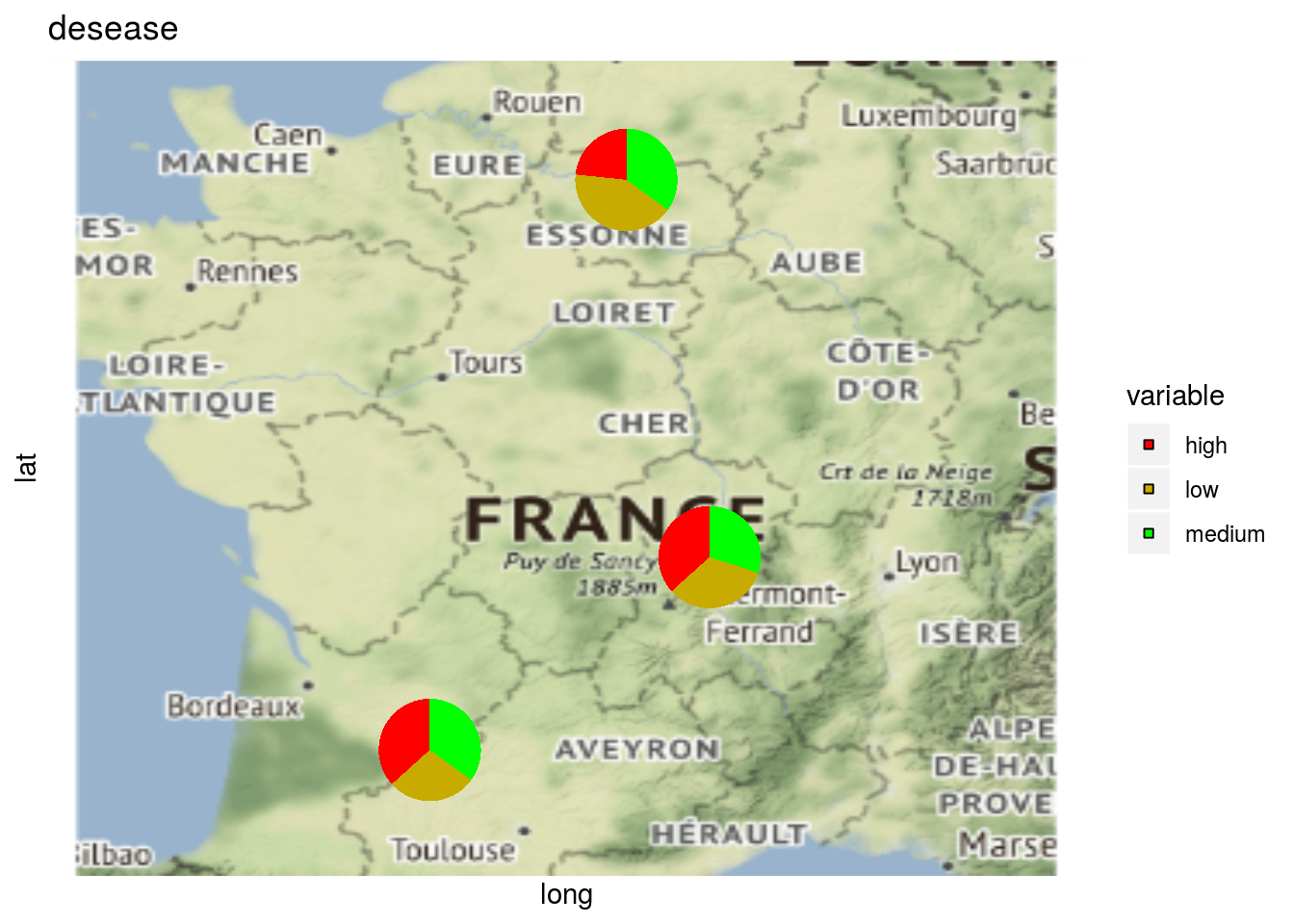## 3.4 Describe the data

Once the data have been collected, a first step is to describe them with plot(). Seven types of plot, through the plot_type argument are possible:

• presence abscence matrix that represent the combinaison of germplasm $$\times$$ location
• histogramm
• barplot, where sd error are displayed
• boxplot
• interaction
• biplot
• raster
• map

Then you must choose which factor to represent on the x axis (x_axis argument), the factor to display in color (in_col argument), and of course the variables to describe (vec_variables argument).

It is possible to tune the number of factor displayed (nb_parameters_per_plot_x_axis and nb_parameters_per_plot_in_col arguments) and the size of the labels regarding biplot and radar (labels_on and labels_size arguments).

Note that descriptive plots can be done based on version within the data set. See section 3.8 formore details.

### 3.4.1 Format the data

Get two data set to look at some examples

data("data_model_GxE")
data_model_GxE = format_data_PPBstats(data_model_GxE, type = "data_agro")
## data has been formated for PPBstats functions.
data("data_model_bh_GxE")
data_model_bh_GxE = format_data_PPBstats(data_model_bh_GxE, type = "data_agro")
## Warning in format_data_PPBstats.data_agro(data): Column "long" is needed to
## get map and not present in data.
## Warning in format_data_PPBstats.data_agro(data): Column "lat" is needed to
## get map and not present in data.
## data has been formated for PPBstats functions.

### 3.4.2 presence abscence matrix

The presence absence matrix may be different from experimental design planned because of NA. The plot represents the presence/absence matrix of G $$\times$$ E combinations.

p = plot(
data_model_GxE, plot_type = "pam",
vec_variables = c("y1", "y2")
)
names(p)
##  "y1" "y2"
p$y1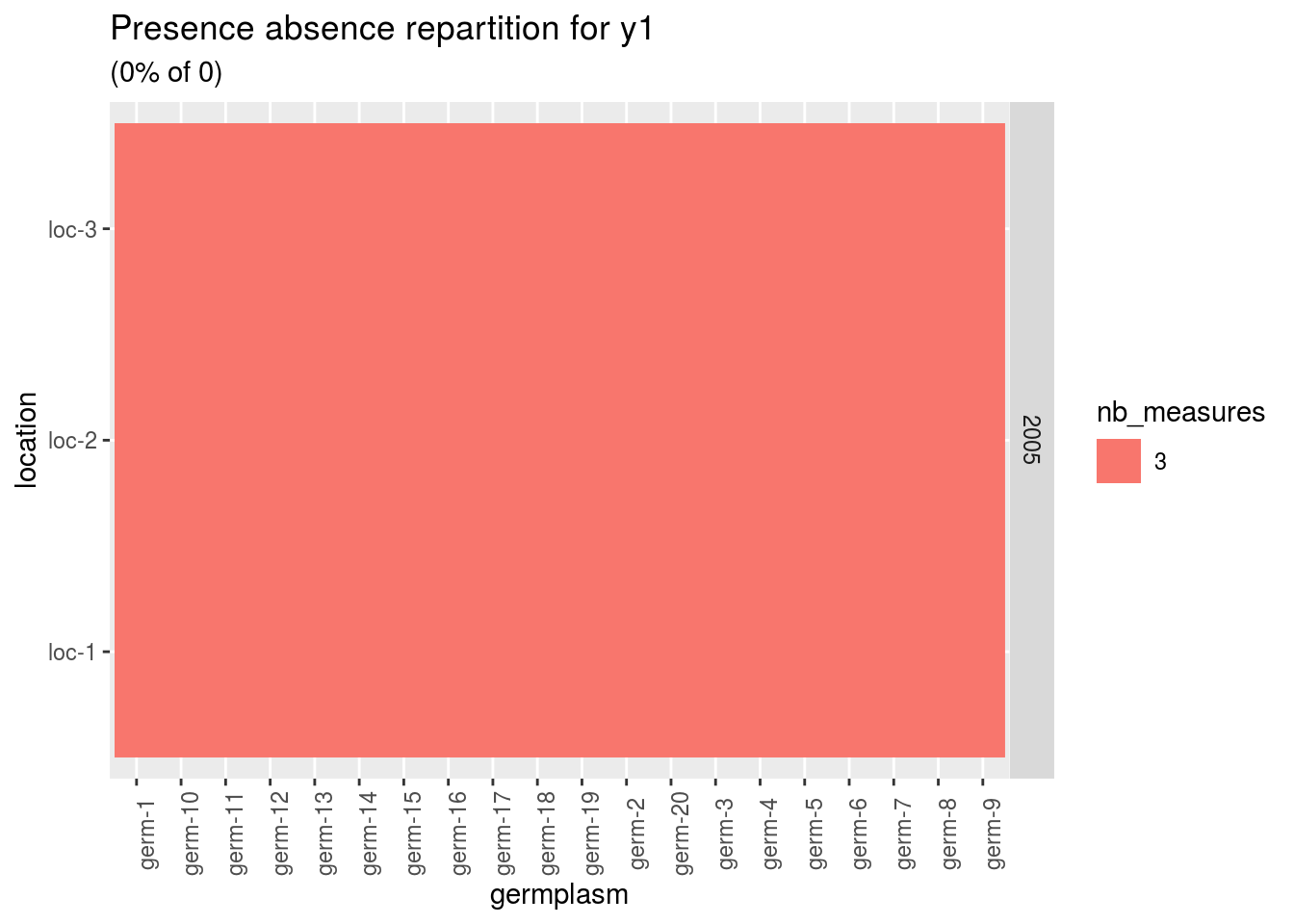A score of 3 is for a given germplasm replicated three times in a given environement. p = plot( data_model_bh_GxE, plot_type = "pam", vec_variables = c("y1", "y2") ) p$y1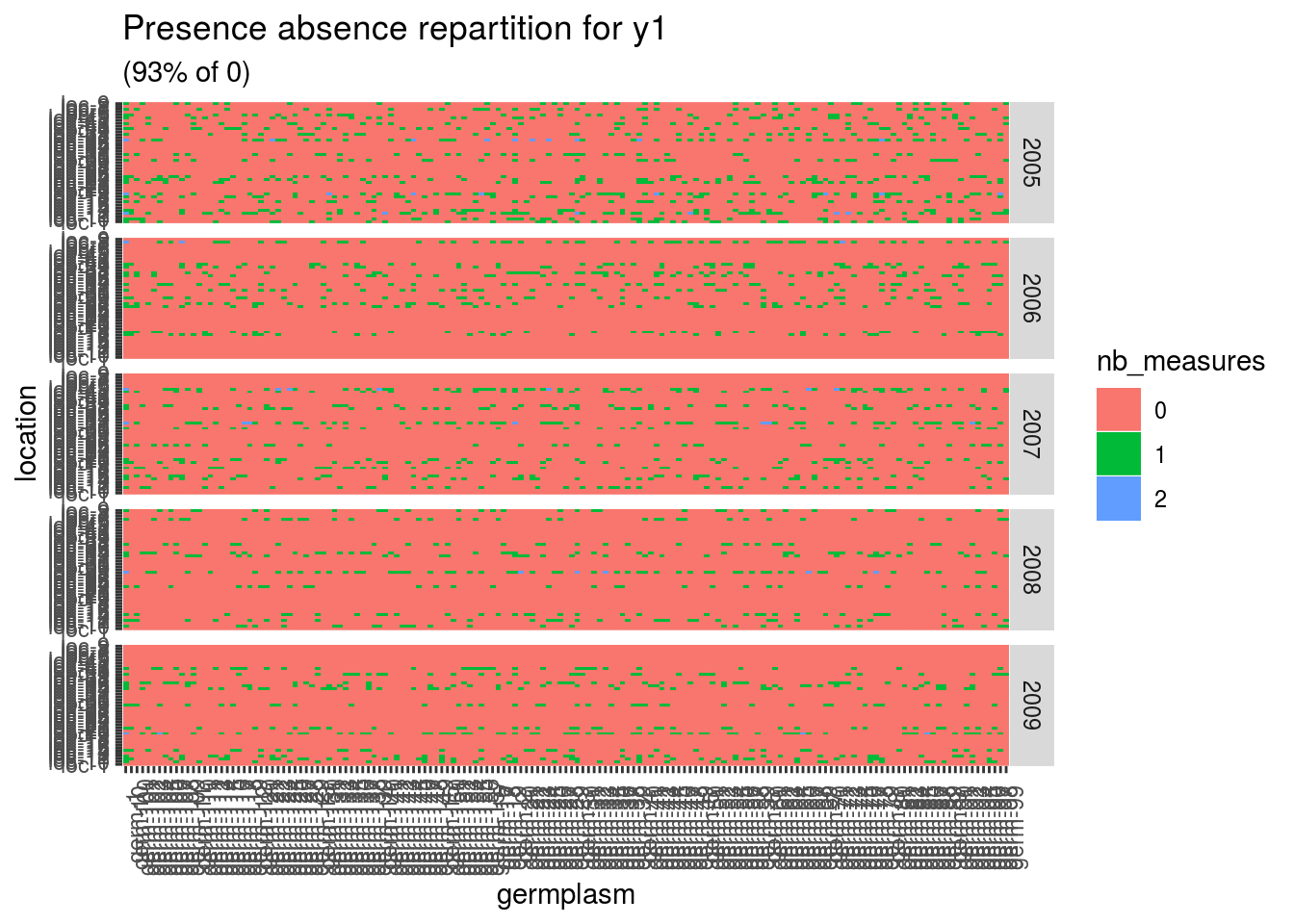Here there are lots of 0 meaning that a lot of germplasm are no in at least two locations. A score of 1 is for a given germplasm in a given location. A score of 2 is for a given germplasm replicated twice in a given location.

### 3.4.3 histogramm

p = plot(
data_model_GxE, plot_type = "histogramm",
vec_variables = c("y1", "y2")
)
p$y1 ##$-NA|-NA
## stat_bin() using bins = 30. Pick better value with binwidth.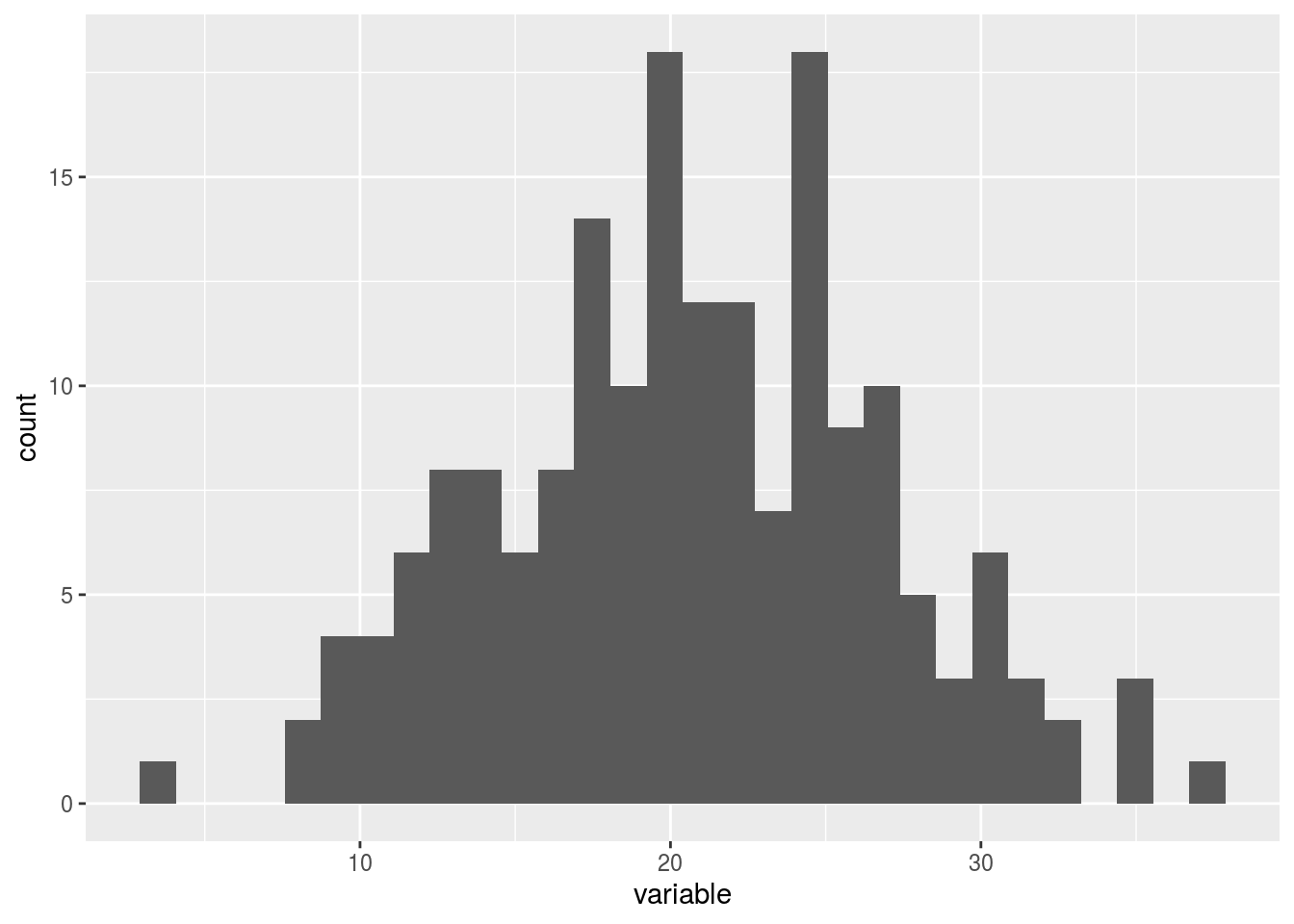### 3.4.4 barplot

p = plot(
data_model_GxE, plot_type = "barplot",
vec_variables = c("y1", "y2"),
x_axis = "germplasm"
)

Note that for each element of the following list, there are as many graph as needed with nb_parameters_per_x_axis parameters per graph.

names(p$y1) ##  "germplasm-1|-NA" "germplasm-2|-NA" "germplasm-3|-NA" "germplasm-4|-NA" p$y1$germplasm-1|-NA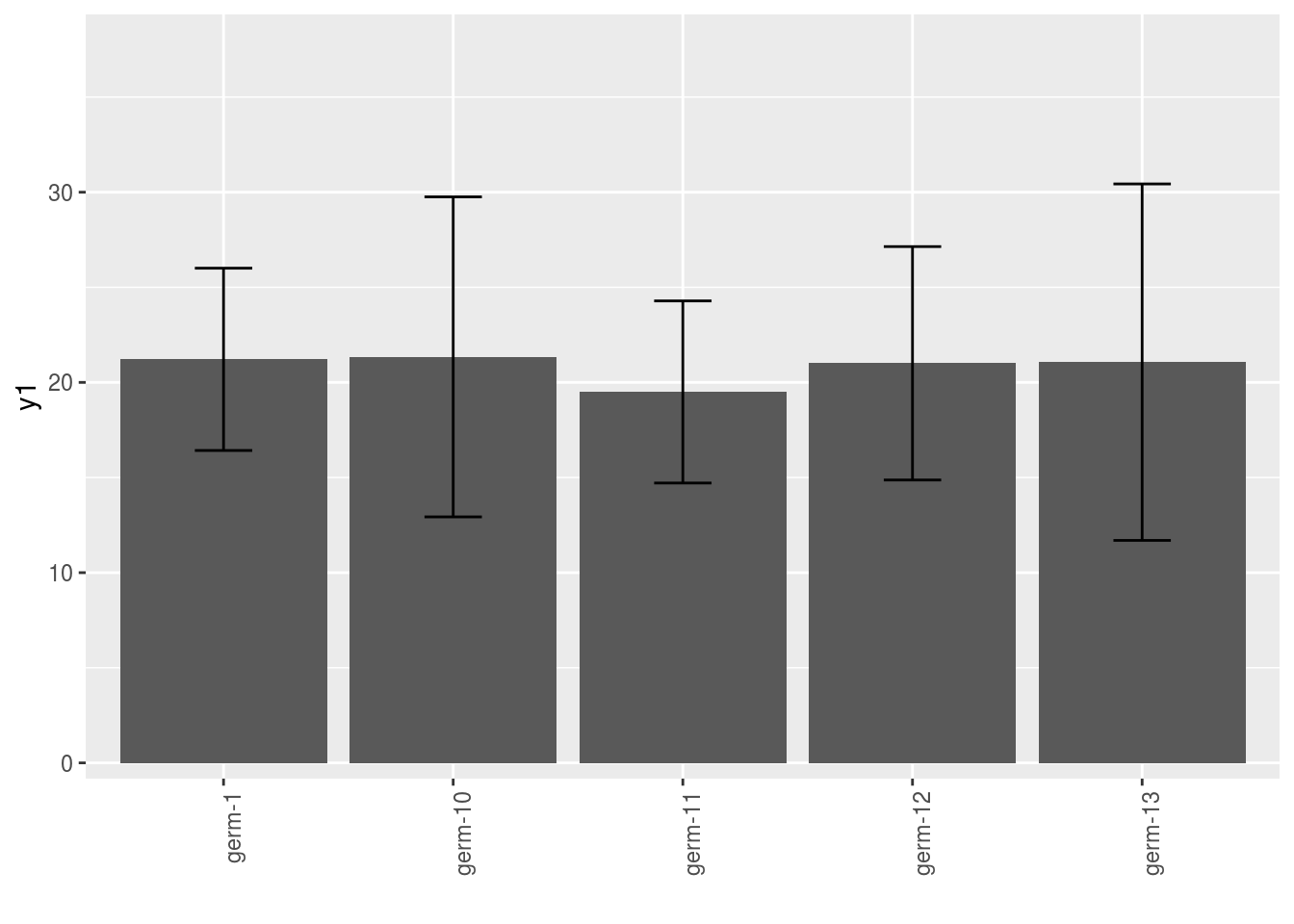p = plot( data_model_GxE, plot_type = "barplot", vec_variables = c("y1", "y2"), x_axis = "germplasm", in_col = "location" ) Note that for each element of the following list, there are as many graph as needed with nb_parameters_per_x_axis and nb_parameters_per_in_col parameters per graph. names(p$y1)
##  "germplasm-1|location-1" "germplasm-2|location-1"
##  "germplasm-3|location-1" "germplasm-4|location-1"
p$y1$germplasm-1|location-1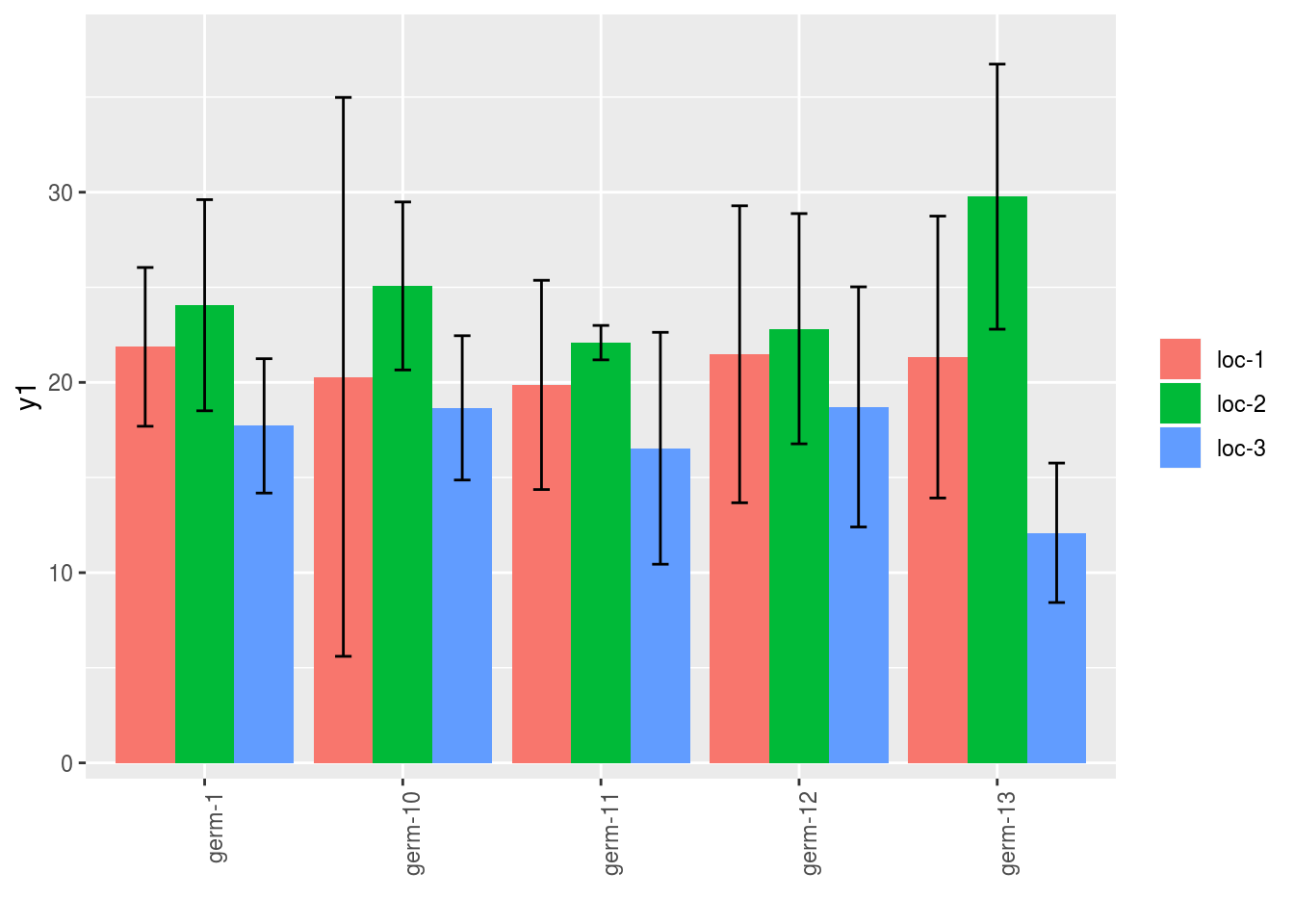### 3.4.5 boxplot

p = plot(
data_model_GxE, plot_type = "boxplot",
vec_variables = c("y1", "y2"),
x_axis = "germplasm"
)

Note that for each element of the following list, there are as many graph as needed with nb_parameters_per_x_axis parameters per graph.

names(p$y1) ##  "germplasm-1|-NA" "germplasm-2|-NA" "germplasm-3|-NA" "germplasm-4|-NA" p$y1$germplasm-1|-NA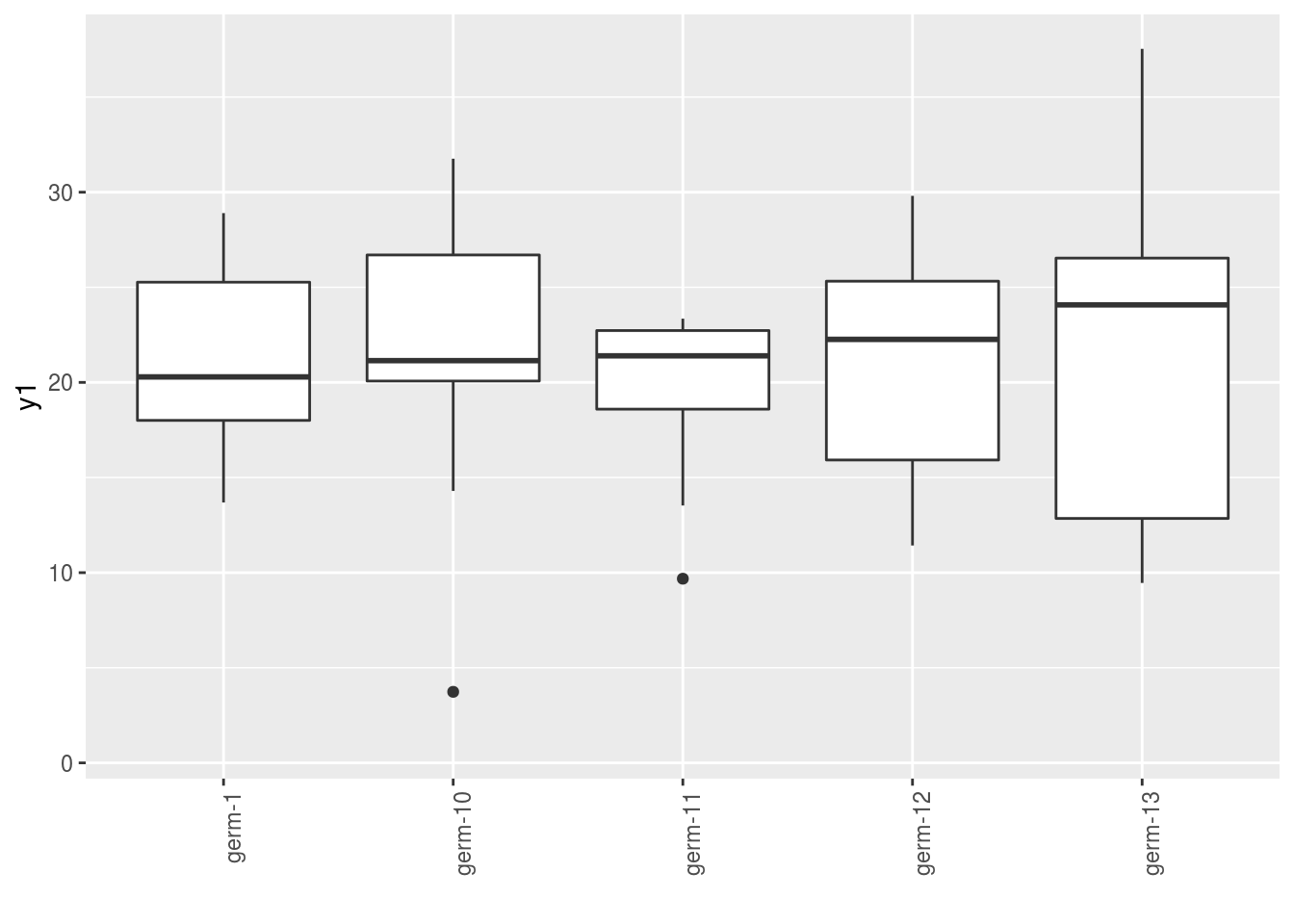p = plot( data_model_GxE, plot_type = "boxplot", vec_variables = c("y1", "y2"), x_axis = "germplasm", in_col = "location" ) Note that for each element of the following list, there are as many graph as needed with nb_parameters_per_x_axis and nb_parameters_per_in_col parameters per graph. names(p$y1)
##  "germplasm-1|location-1" "germplasm-2|location-1"
##  "germplasm-3|location-1" "germplasm-4|location-1"
p$y1$germplasm-1|location-1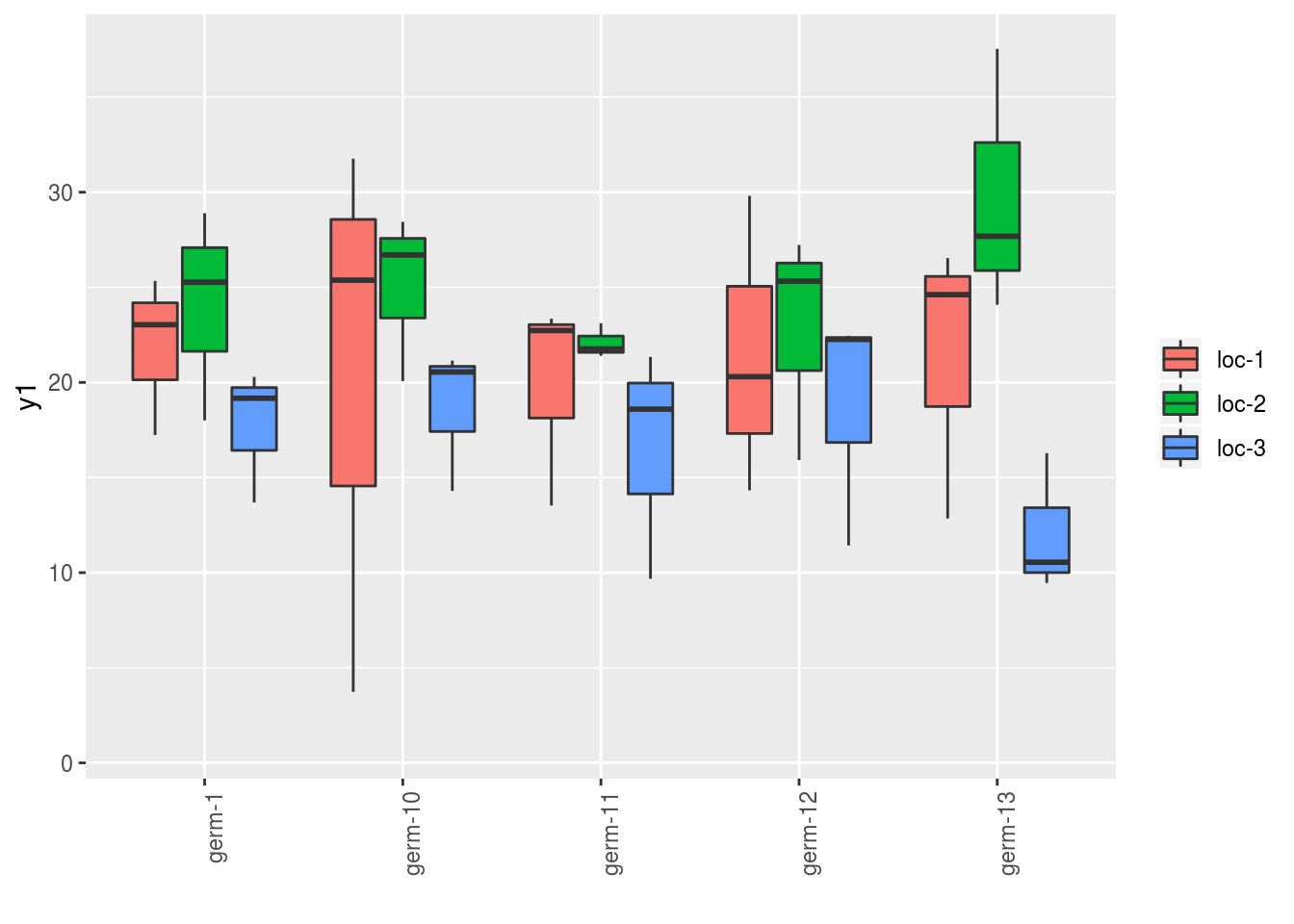### 3.4.6 interaction

p = plot(
data_model_GxE, plot_type = "interaction",
vec_variables = c("y1", "y2"),
x_axis = "germplasm",
in_col = "location"
)

Note that for each element of the following list, there are as many graph as needed with nb_parameters_per_x_axis and nb_parameters_per_in_col parameters per graph.

names(p$y1) ##  "germplasm-1|location-1" "germplasm-2|location-1" ##  "germplasm-3|location-1" "germplasm-4|location-1" p$y1$germplasm-1|location-1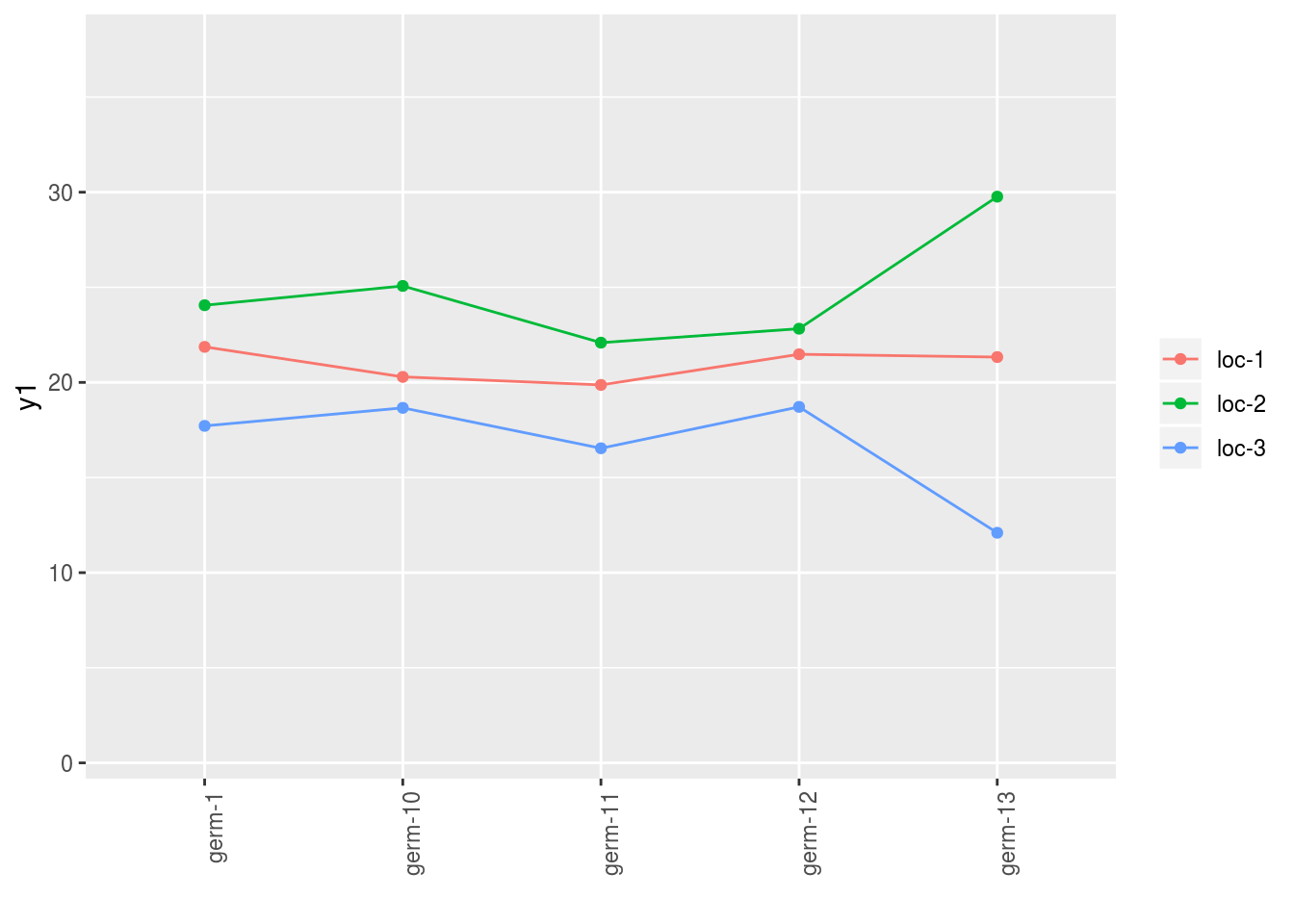It is also possible to have on the x_axis the date in julian day that have been automatically calculated from format_data_PPBstats(). Note that this is possible only for plot_type = "histogramm", "barplot", "boxplot" and "interaction". p = plot( data_model_GxE, plot_type = "interaction", vec_variables = c("y1", "y2"), x_axis = "date_julian", in_col = "location" ) ## Warning in plot_descriptive_data(x, plot_type, x_axis, in_col, ## vec_variables, : x_axis = "date_julian" is a special feature that will ## display julian day for a given variable automatically calculated from ## format_data_PPBstats(). p$y1$y1$date_julian-1|location-1
## geom_path: Each group consists of only one observation. Do you need to
## adjust the group aesthetic?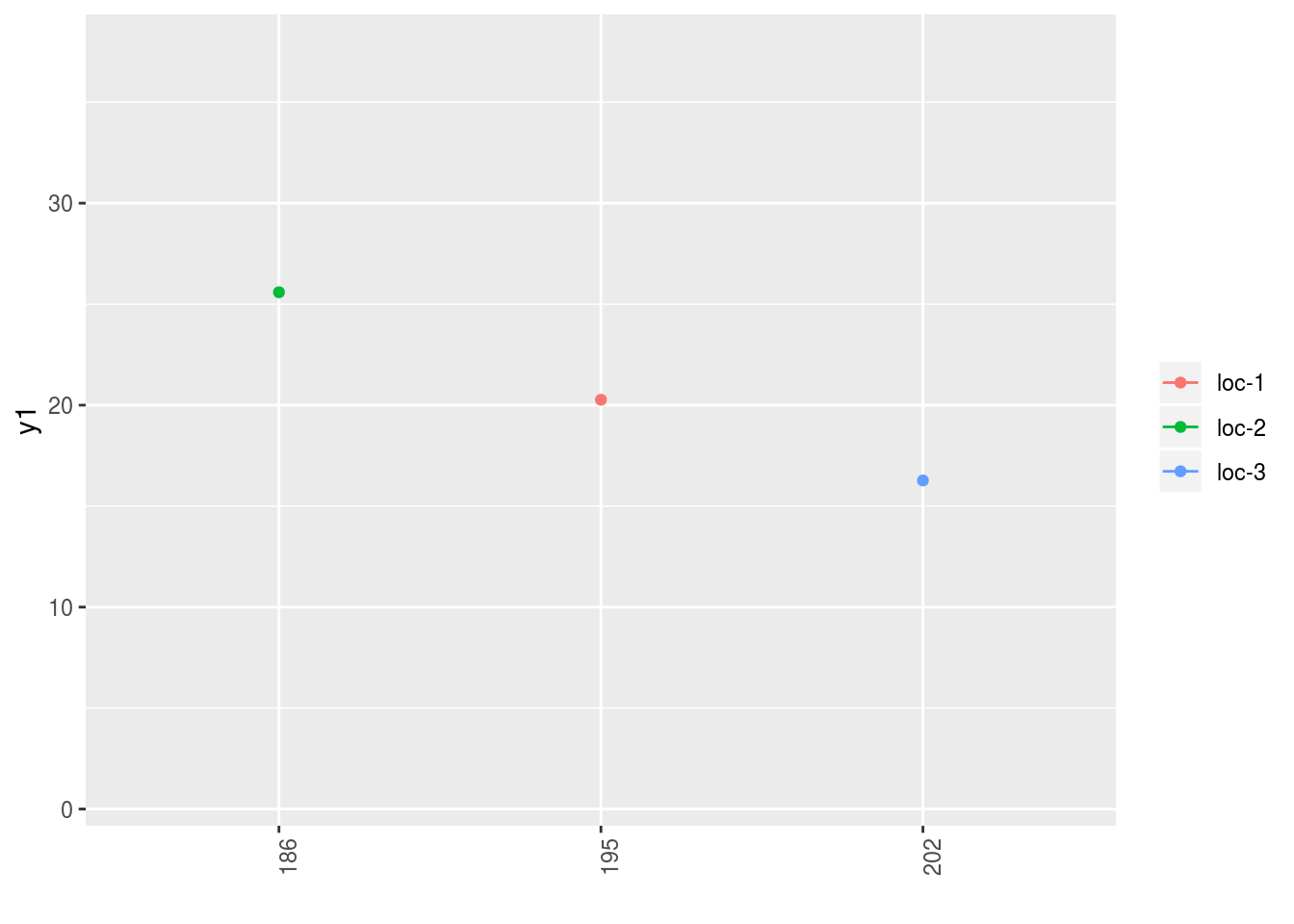### 3.4.7 biplot

p = plot(
data_model_GxE, plot_type = "biplot",
vec_variables = c("y1", "y2", "y3"),
in_col = "germplasm", labels_on = "germplasm"
)

The name of the list correspond to the pairs of variables displayed. Note that for each element of the following list, there are as many graph as needed with nb_parameters_per_in_col parameters per graph.

names(p)
##  "y1 - y2" "y1 - y3" "y2 - y3"
p$y1 - y2$-NA|germplasm-1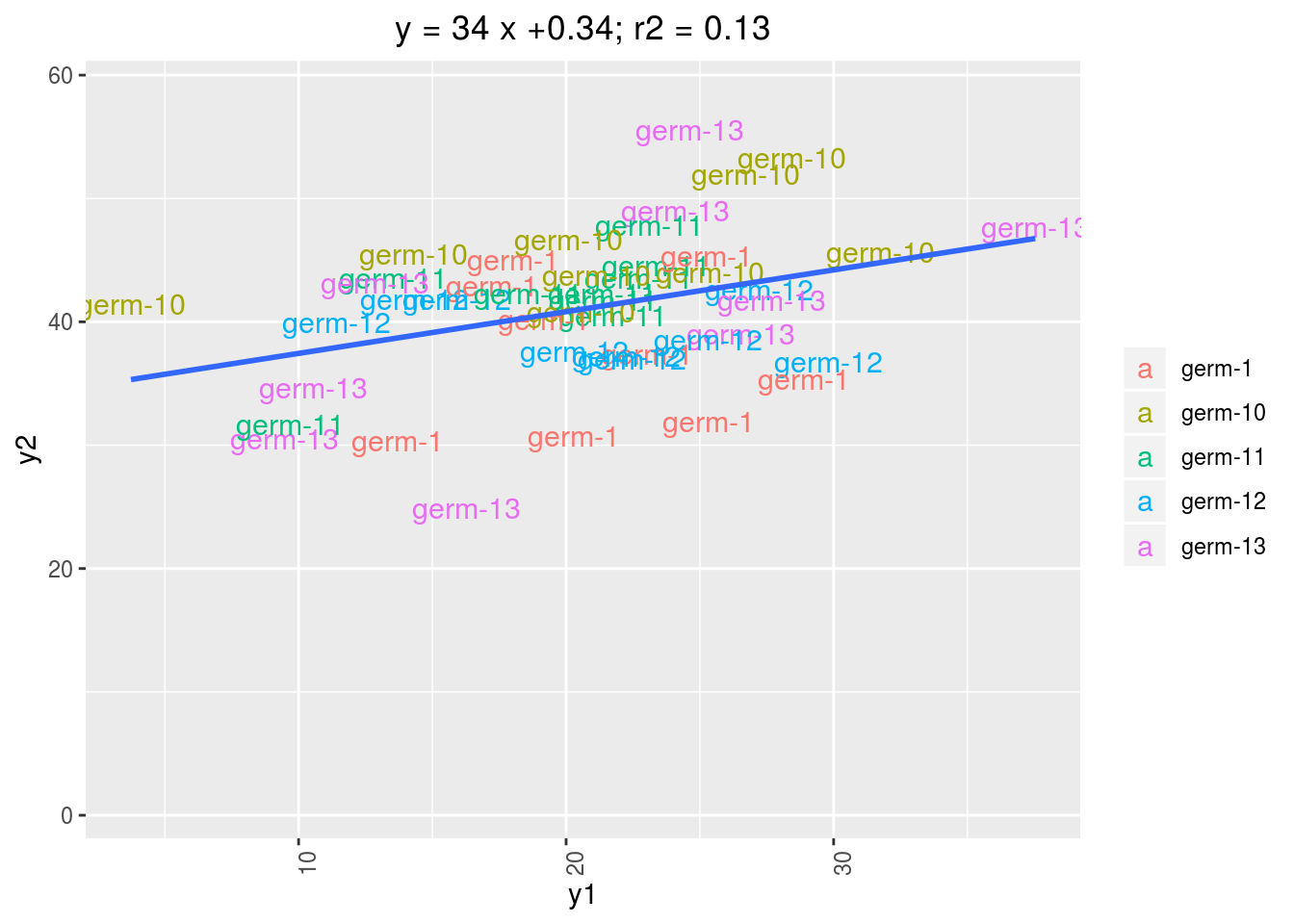p = plot(
vec_variables = c("y1", "y2", "y3"),
in_col = "location"
)
p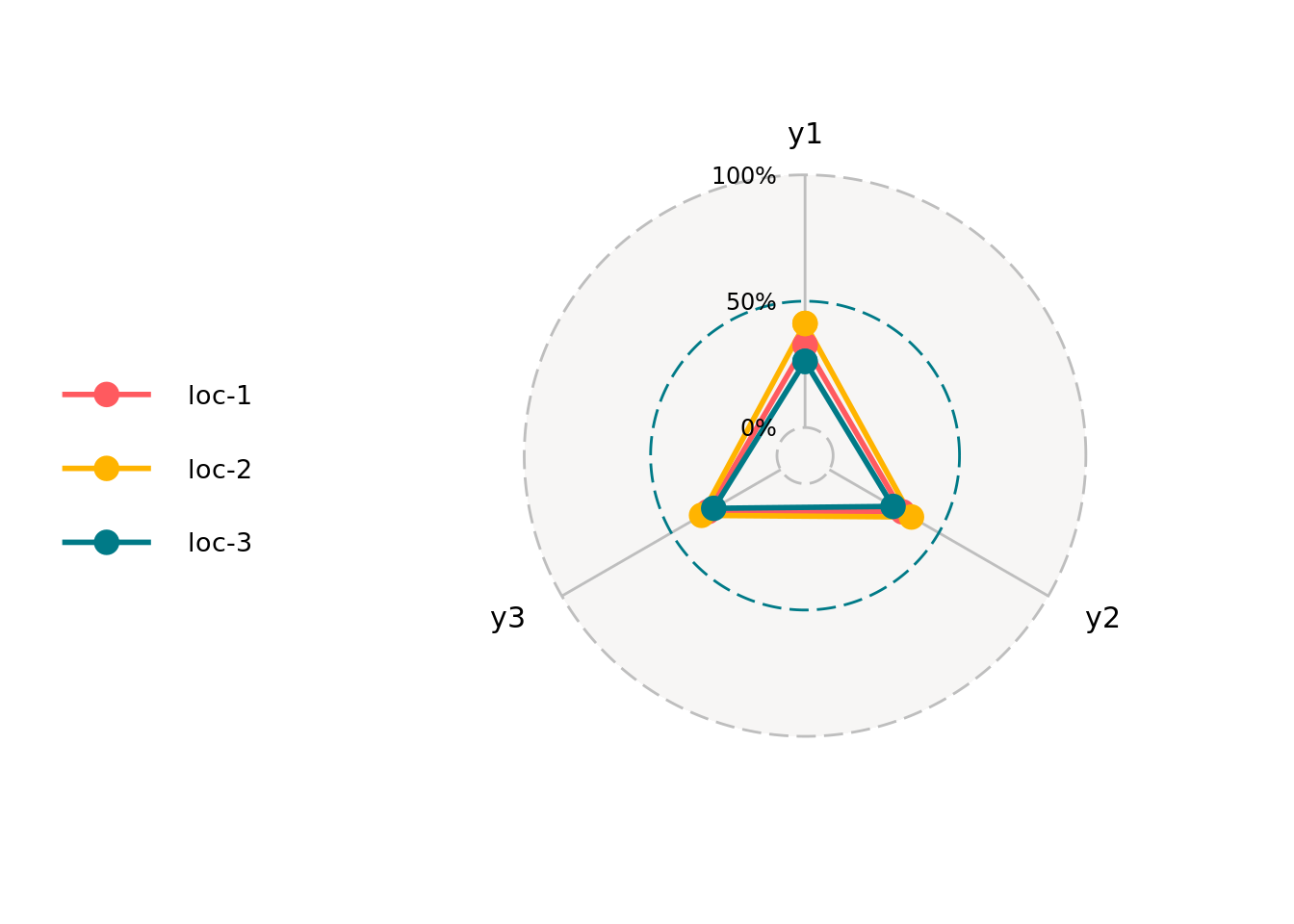### 3.4.9 raster

Raster plot can be done for factor variables. Note than when there are no single value for a given x_axis, colums block, X and Y are added in order to have single value.

p = plot(
data_model_GxE,
plot_type = "raster",
vec_variables = c("desease", "vigor"),
x_axis = "germplasm"
)
## Warning in fun_raster(data, vec_variables, x_axis,
## nb_parameters_per_plot_x_axis): There are no single value for each x_axis,
## therefore block, X and Y colums have been added in order to have single
## value.
p$germplasm-block-X-Y-9|-NA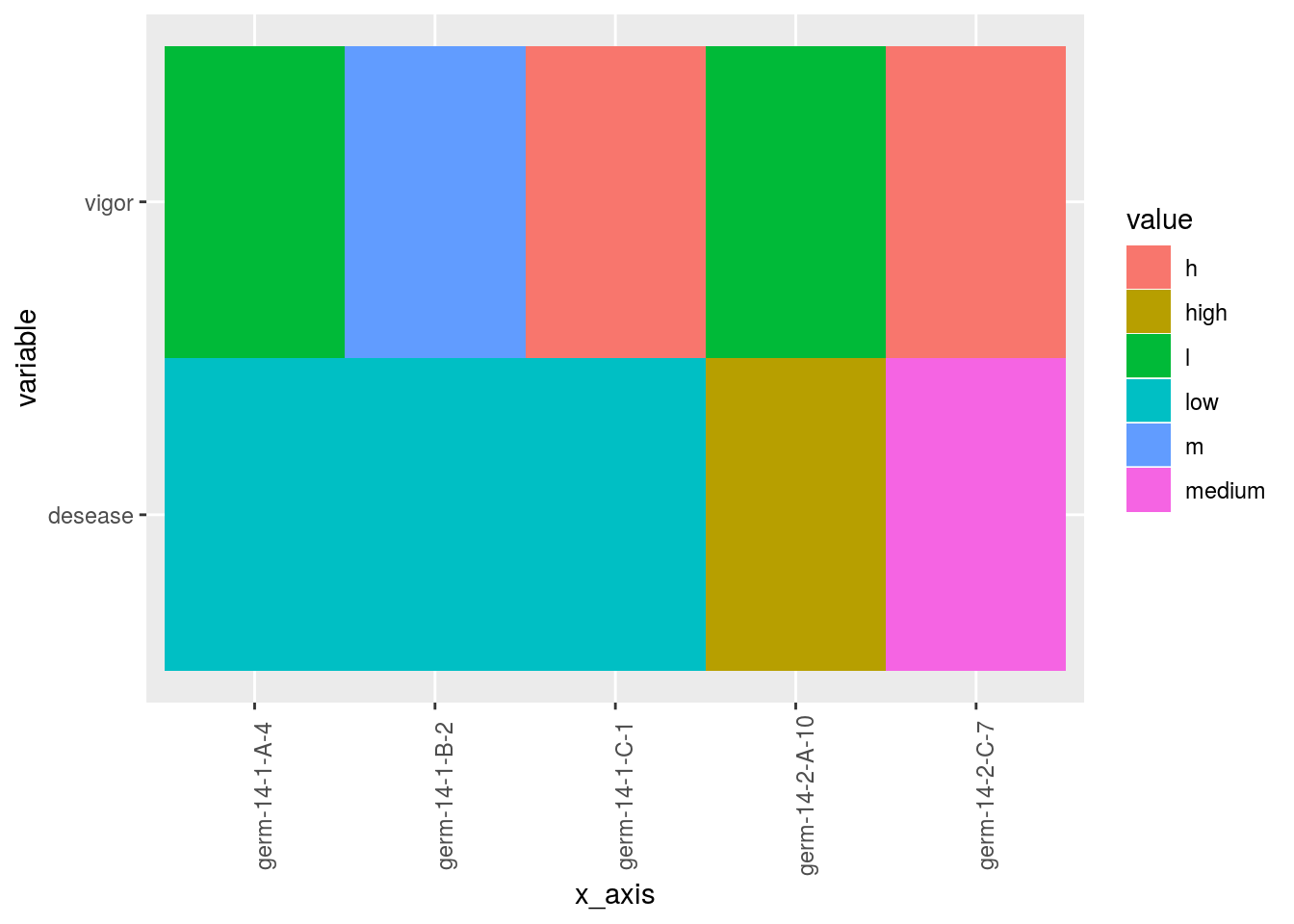### 3.4.10 map You can display map with location if you have data with latitude and longitude for each location. When using map, do not forget to use credit : Map tiles by Stamen Design, under CC BY 3.0. Data by OpenStreetMap, under ODbL. p = plot( data_model_GxE, plot_type = "map", labels_on = "location" ) p$map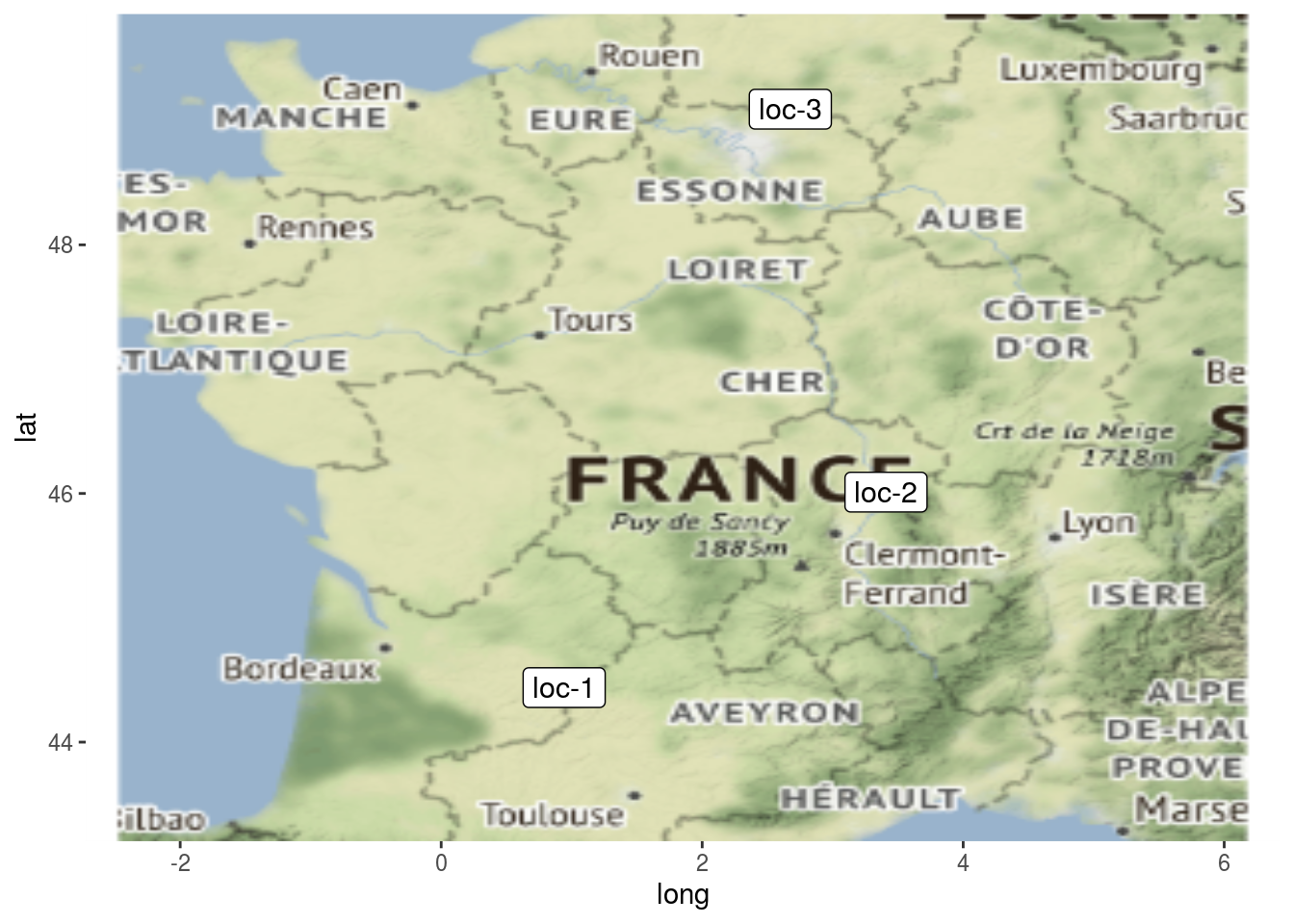and add pies for a given variables

p = plot(
data_model_GxE, vec_variables = c("y1", "desease"),
plot_type = "map"
)
p$pies_on_map_y1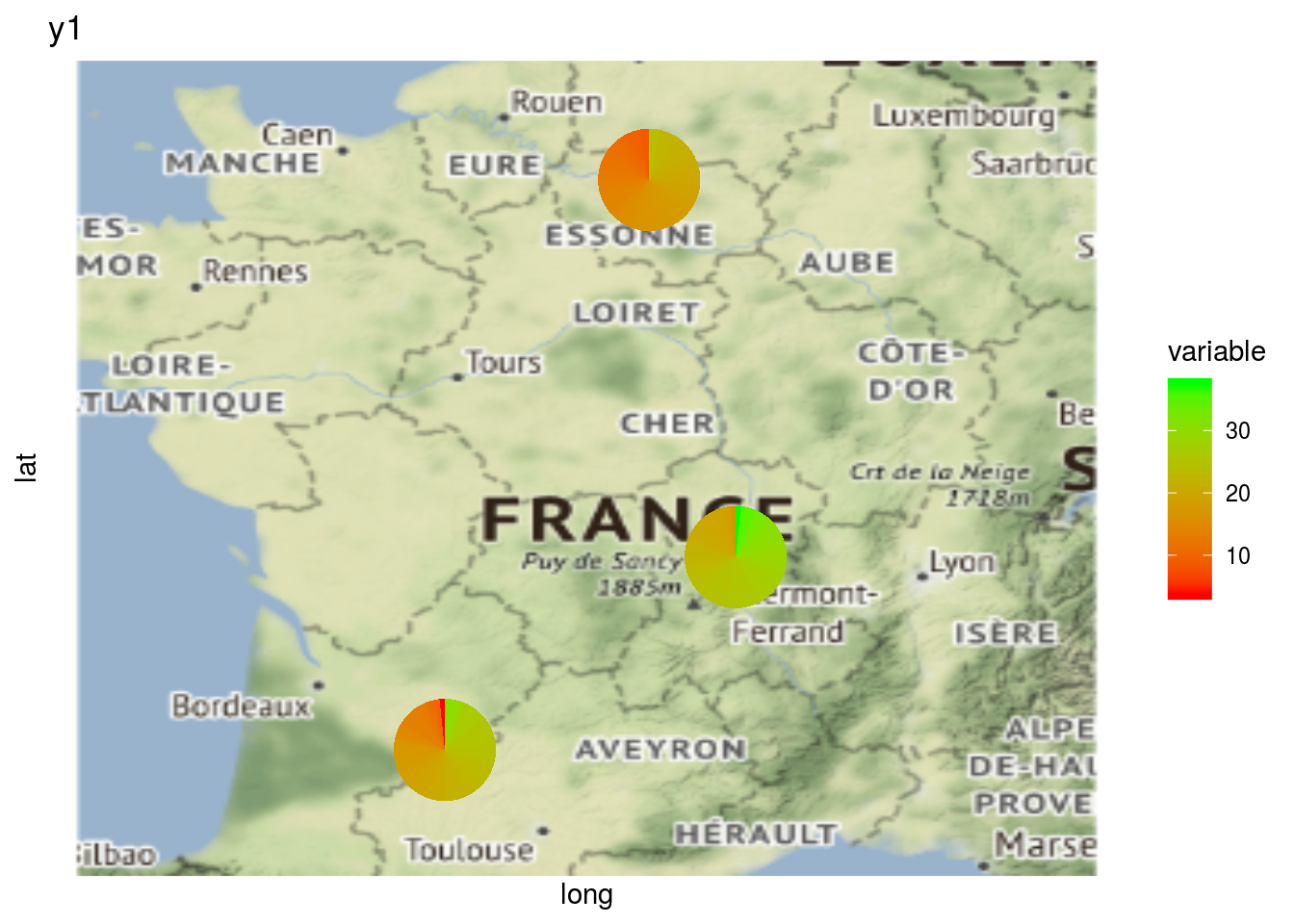p$pies_on_map_desease